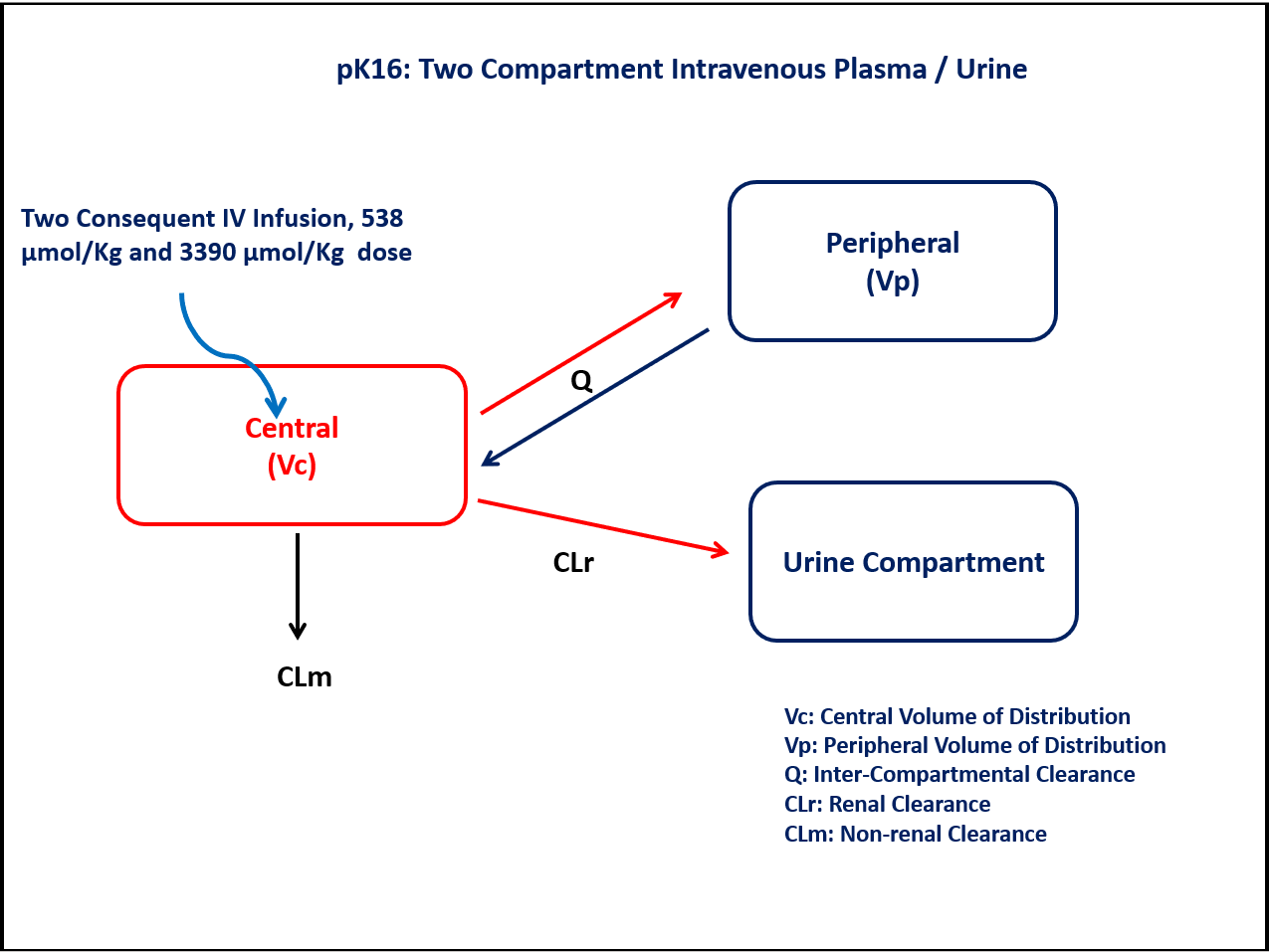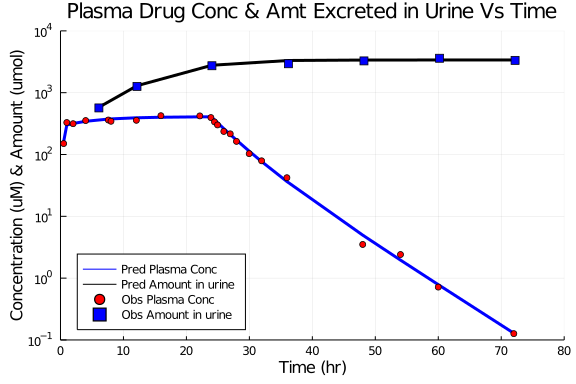# Exercise PK16 - Two compartment intravenous plasma/urine

### Background

• Structural Model - Two Compartment model with first order elimination

• Route of Administration - Intravenous infusion (Multiple-dose)

• Dosage Regimen - 538 µmol/kg followed by 3390 µmol/kg

• Number of Subjects - 1### Learning Outcome

This exercise explains simultaneous analysis of plasma and urine data using Two-compartment model with additional Urinary compartment accounting for amount of drug excreted in Urine.

### Objectives

In this exercise, you will learn how to build a two compartment model and to simulate the data for a single subject based on the given dosage regimen.

### Libraries

call the "necessary" libraries to get started.

using Pumas
using Plots
using CSV
using StatsPlots
using Random


### Model

Two-compartment model with one Central and one Peripheral compartment will help in understanding the plasma concentration. A separate Urine compartment accounts for the fraction (amount) of drug excreted in Urine.

pk_16             = @model begin
@param begin
tvclr       ∈ RealDomain(lower=0)
tvclm       ∈ RealDomain(lower=0)
tvvc        ∈ RealDomain(lower=0)
tvvp        ∈ RealDomain(lower=0)
tvq         ∈ RealDomain(lower=0)
Ω           ∈ PDiagDomain(5)
σ²₁_prop    ∈ RealDomain(lower=0)
σ²₂_prop    ∈ RealDomain(lower=0)
end

@random begin
η           ~ MvNormal(Ω)
end

@pre begin
CLr         = tvclr*exp(η)
CLm         = tvclm*exp(η)
Vc          = tvvc*exp(η)
Vp          = tvvp*exp(η)
Q           = tvq*exp(η)
CL          = CLr+CLm
Vss         = Vc+Vp
t12         = 0.693*Vss/CL
end

@dynamics begin
Central'    = - (CLr/Vc)*Central - (CLm/Vc)*Central + (Q/Vp)*Peripheral - (Q/Vc)*Central
Peripheral' =   (Q/Vc)*Central - (Q/Vp)*Peripheral
Urine'      =   (CLr/Vc)*Central
end

@derived begin
cp_plasma   = @. Central/Vc
dv_plasma   ~ @. Normal(cp_plasma, sqrt(cp_plasma^2*σ²₁_prop))
cp_urine    = @. Urine
dv_urine    ~ @. Normal(cp_urine, sqrt(cp_urine^2*σ²₂_prop))
end
end

PumasModel
Parameters: tvclr, tvclm, tvvc, tvvp, tvq, Ω, σ²₁_prop, σ²₂_prop
Random effects: η
Covariates:
Dynamical variables: Central, Peripheral, Urine
Derived: cp_plasma, dv_plasma, cp_urine, dv_urine
Observed: cp_plasma, dv_plasma, cp_urine, dv_urine


### Parameters

• CLr - Renal Clearance (L/hr/kg)

• CLm - Non-renal Clearance (L/hr/kg)

• Vc - Volume of Central Compartment (L/kg)

• Vp - Volume of Peripheral Compartment (L/kg)

• Q - Inter-compartmental Clearance (L/hr/kg)

• Ω - Between Subject Variability

• σ²₁ - Residual error 1 (for plasma conc)

• σ²₂ - Residual error 2 (for amount excreted in urine)

Derived Parameters:

• CL - Total Clearance (L/hr/kg)

• Vss - Volume of distribution at steady state (L/kg)

• t1/2 - Half life (hr)

Typical value (tv) estimates for individual parameters

param = ( tvclm    = 0.05,
tvclr    = 0.31,
tvvc     = 1.6,
tvvp     = 0.16,
tvq      = 0.03,
Ω        = Diagonal([0.0,0.0,0.0,0.0,0.0]),
σ²₁_prop = 0.01,
σ²₂_prop = 0.01)

(tvclm = 0.05, tvclr = 0.31, tvvc = 1.6, tvvp = 0.16, tvq = 0.03, Ω = [0.0
0.0 … 0.0 0.0; 0.0 0.0 … 0.0 0.0; … ; 0.0 0.0 … 0.0 0.0; 0.0 0.0 … 0.0 0.0]
, σ²₁_prop = 0.01, σ²₂_prop = 0.01)


### DosageRegimen

• The subject (male dog) received two consecutive intravenous infusions of a drug.

• The subject received initial dose of 538 µmol/kg as from time 0 to 0.983 hr (rate= 547.304) followed by 3390 µmol/kg from time 0.983 to 23.95 hr (rate=147.603).

• Total infused dose: 3928 µmol/kg.

ev1  = DosageRegimen([538,3390], time = [0,0.983], cmt = [1,1], rate = [547.304,147.603])
sub1 = Subject(id=1, events=ev1)

Subject
ID: 1
Events: 4


### Simulation

To simulate the following for the above subject with specific observation time points.

1. Plasma concentration.

2. Amount excreted unchanged in Urine.

Random.seed!(123)
sim_sub1 = simobs(pk_16,sub1,param,obstimes = [0.5,1,2,4,6.1,7.6,8.02,12.05,12.15,15.95,22.13,23.89,24.05,24.46,24.94,25.94,26.96,27.95,29.97,31.94,35.96,36.2,48,48.2,54,60,60.2,72,72.2])


### DataFrame & Plot

Create a dataframe of the simulated data.

df1       = DataFrame(sim_sub1)
df_plasma = filter(x -> x.time in [0.5,1,2,4,7.6,8.02,12.05,15.95,22.13,23.89,24.46,24.94,25.94,26.96,27.95,29.97,31.94,35.96,48,54,60,72], df1)
df_urine  = filter(x -> x.time in [6.1, 12.15, 24.05, 36.2, 48.2, 60.2, 72.2], df1)


To plot the simulated plasma concentration data and amount of drug excreted in urine in a single plot.

@df df_plasma plot(:time, :cp_plasma, yaxis=:log,
label="Pred Plasma Conc", xlabel="Time (hr)", ylabel= "Concentration (uM) & Amount (umol)",
title= "Plasma Drug Conc & Amt Excreted in Urine Vs Time",
color = "blue", linewidth=3, legend=:bottomleft,
xticks=[0,10,20,30,40,50,60,70,80], yticks=[0.1,0,1,10,100,1000,10000], ylims=(0.1,10000), xlims=(0,80))
@df df_urine plot!(:time, :cp_urine, yaxis=:log,
label="Pred Amount in urine", color= "black", linewidth=3)
@df df_plasma scatter!(:time, :dv_plasma, yaxis=:log,
label="Obs Plasma Conc", color= "red", markershape=:circle)
@df df_urine scatter!(:time, :dv_urine, yaxis=:log,
label="Obs Amount in urine", color= "blue", markershape=:square)